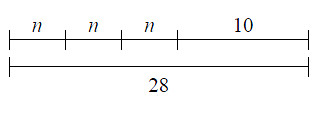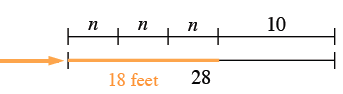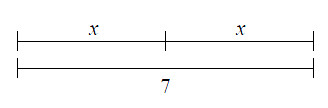### Home > MC1 > Chapter 3 > Lesson 3.2.1 > Problem3-29

3-29.

Each of the diagrams below represents a tightrope routine for an acrobat. For each diagram,

• Describe what the diagram shows about the routine and the length of the tightrope.

• Figure out the length of the acrobat's trick.

1.This is similar to writing an expression for an acrobat's routine. Think of the tricks as being numerical lengths of the rope.

The acrobat performs same trick three times and then walks $10$ feet to get to the end of the $28$ foot tight rope.

The trick travels $6$ feet.

First, describe the routine based on the diagram.

Next, determine how far each trick travels. Looking at the tightropes, the $3$ $n$'s match up with $18$ feet. Since $3$ unknown, but equal, lengths combine to make $18$ feet, you know that each length is $6$ feet.2.Follow the same steps as in part (a). Remember to try the problem on your own before checking the answer.